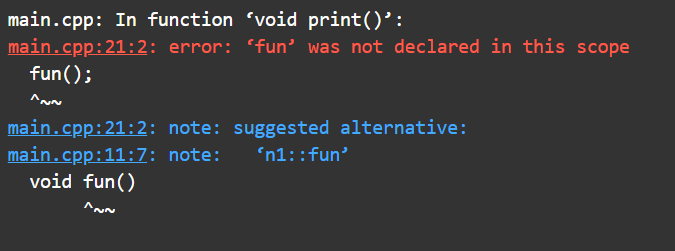# Why it is important to write “using namespace std” in C++ program?

• Difficulty Level : Easy
• Last Updated : 22 Nov, 2021

In this article, we will discuss the use of “using namespace std” in the C++ program.

Need of namespace:

• As the same name can’t be given to multiple variables, functions, classes, etc. in the same scope.
• So to overcome this situation namespace is introduced.

Program 1:

Below is the C++ program illustrating the use of namespace with the same name of function and variables:

## C++

 `// C++ program to illustrate the use``// of namespace with same name of``// function and variables``#include ``using` `namespace` `std;` `// Namespace n1``namespace` `n1 {``int` `x = 2;` `// Function to display the message``// for namespace n1``void` `fun()``{``    ``cout << ``"This is fun() of n1"``         ``<< endl;``}``}` `// Namespace n2``namespace` `n2 {` `int` `x = 5;` `// Function to display the message``// for namespace n2``void` `fun()``{``    ``cout << ``"This is fun() of n2"``         ``<< endl;``}``}` `// Driver Code``int` `main()``{``    ``// The methods and variables called``    ``// using scope resolution(::)``    ``cout << n1::x << endl;` `    ``// Function call``    ``n1::fun();` `    ``cout << n2::x << endl;` `    ``// Function ca;;``    ``n2::fun();` `    ``return` `0;``}`

Output:

```2
This is fun() of n1
5
This is fun() of n2```

Explanation:

• In the above example program, both n1 and n2 have a variable and function of the same name x and fun() respectively.
• The namespace is used to decrease or limit the scope of any variable or function.
• As in the above code variable x and method fun() were limited to namespaces n1 and n2. Thus, their scope was not outside the n1 or n2.
• Every time using the scope resolution operator (::) in a variable or a function defined is not required, it can be solved with “using” directive.
• The using directive means to include the whole code written in the namespace in the closing scope.

Program 2:

Below is the C++ program demonstrating the use of the “using” directive:

## C++

 `// C++ program to demonstrate the use``// of "using" directive``#include ``using` `namespace` `std;` `// Namespace n1``namespace` `n1 {``int` `x = 2;``void` `fun()``{``    ``cout << ``"This is fun() of n1"``         ``<< endl;``}``}` `// Namespace is included``using` `namespace` `n1;` `// Driver Code``int` `main()``{``    ``cout << x << endl;` `    ``// Function Call``    ``fun();` `    ``return` `0;``}`

Output:

```2
This is fun() of n1```

Explanation:

• In the above program, after writing “using namespace n1“, there is no need to use the scope resolution for utilizing the members of n1.
• It can be interpreted as “using” copies of the code written in the namespace to the scope in which it has been written.

If “using namespace n1” is written inside the main() and tries to use the members (fun() and x in this case) in the different functions it would give a compile-time error.

Program 3:

Below is the C++ program illustrating the use of “using namespace” inside main() function:

## C++

 `// C++ program illustrating the use``// of "using namespace" inside main()` `#include ``using` `namespace` `std;` `// Namespace n1``namespace` `n1 {``int` `x = 2;``void` `fun()``{``    ``cout << ``"This is fun() of n1"``         ``<< endl;``}``}` `// Function calling function``void` `print()``{``    ``// Gives error, used without ::``    ``fun();``}` `// Driver Code``int` `main()``{``    ``// Namespace inside main``    ``using` `namespace` `n1;` `    ``cout << x << endl;` `    ``// Function Call``    ``fun();` `    ``return` `0;``}`

Output:Explanation:

• It is known that “std” (abbreviation for the standard) is a namespace whose members are used in the program.
• So the members of the “std” namespace are cout, cin, endl, etc.
• This namespace is present in the iostream.h header file.
• Below is the code snippet in C++ showing content written inside iostream.h:

## C++

 `// Code written in the iostream.h file` `namespace` `std {``ostream cout;``i0stream cin;``// and some more code``}`

Explanation:

• Now when cout<<“GeeksforGeeks”; is written, the compiler searches for cout in our program which is kept in the std namespace, so the instruction given to the compiler that if the compiler doesn’t find anything in the current scope, try finding it in the std namespace.
• It is not necessary to write namespaced, simply use scope resolution (::) every time uses the members of std. For example, std::cout, std::cin, std::endl etc.

Program 4:

Below is the C++ program illustrating the use of std:

## C++

 `// C++ program to illustrate``// the use of std``#include ` `// Driver Code``int` `main()``{``    ``int` `x = 10;``    ``std::cout << ``" The value of x is "``              ``<< x << std::endl;``    ``return` `0;``}`

Output:

`The value of x is 10`

Explanation: The output of the program will be the same whether write “using namespace std” or use the scope resolution.

My Personal Notes arrow_drop_up